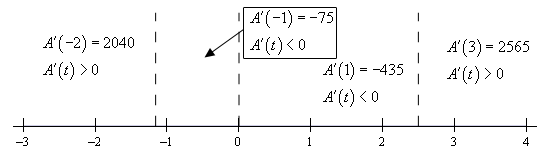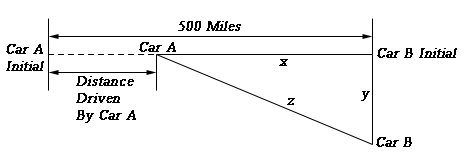Paul's Online Notes
Home / Calculus I / Applications of Derivatives / Rates of Change
Show Mobile Notice Show All Notes Hide All Notes
Mobile Notice
You appear to be on a device with a "narrow" screen width (i.e. you are probably on a mobile phone). Due to the nature of the mathematics on this site it is best views in landscape mode. If your device is not in landscape mode many of the equations will run off the side of your device (should be able to scroll to see them) and some of the menu items will be cut off due to the narrow screen width.

### Section 4-1 : Rates of Change

The purpose of this section is to remind us of one of the more important applications of derivatives. That is the fact that $$f'\left( x \right)$$ represents the rate of change of $$f\left( x \right)$$. This is an application that we repeatedly saw in the previous chapter. Almost every section in the previous chapter contained at least one problem dealing with this application of derivatives. While this application will arise occasionally in this chapter we are going to focus more on other applications in this chapter.

So, to make sure that we don’t forget about this application here is a brief set of examples concentrating on the rate of change application of derivatives. Note that the point of these examples is to remind you of material covered in the previous chapter and not to teach you how to do these kinds of problems. If you don’t recall how to do these kinds of examples you’ll need to go back and review the previous chapter.

Example 1 Determine all the points where the following function is not changing. $g\left( x \right) = 5 - 6x - 10\cos \left( {2x} \right)$
Show Solution

First, we’ll need to take the derivative of the function.

$g'\left( x \right) = - 6 + 20\sin \left( {2x} \right)$

Now, the function will not be changing if the rate of change is zero and so to answer this question we need to determine where the derivative is zero. So, let’s set this equal to zero and solve.

$- 6 + 20\sin \left( {2x} \right) = 0\hspace{0.5in} \Rightarrow \hspace{0.5in}\sin \left( {2x} \right) = \frac{6}{{20}} = 0.3$

The solution to this is then,

\begin{alignat}{4}2x = & 0.3047 + 2\pi n & & \hspace{0.5in}\,\,\,\,{\mbox{OR}}\hspace{0.5in}\,\,\,\, & 2x = & 2.8369 + 2\pi n & & \hspace{0.25in}n = 0, \pm 1, \pm 2, \ldots \\ x = & 0.1524 + \pi n & & \hspace{0.5in}\,\,\,\,{\mbox{OR}}\hspace{0.5in}\,\,\, & x = & 1.4185 + \pi n & & \hspace{0.25in}n = 0, \pm 1, \pm 2, \ldots \end{alignat}

If you don’t recall how to solve trig equations check out the Solving Trig Equations sections in the Review Chapter.

Example 2 Determine where the following function is increasing and decreasing. $A\left( t \right) = 27{t^5} - 45{t^4} - 130{t^3} + 150$
Show Solution

As with the first problem we first need to take the derivative of the function.

$A'\left( t \right) = 135{t^4} - 180{t^3} - 390{t^2} = 15{t^2}\left( {9{t^2} - 12t - 26} \right)$

Next, we need to determine where the function isn’t changing. This is at,

\begin{align*}t & = 0\\ t & = \frac{{12 \pm \sqrt {144 - 4\left( 9 \right)\left( { - 26} \right)} }}{{18}} = \frac{{12 \pm \sqrt {1080} }}{{18}} = \frac{{12 \pm 6\sqrt {30} }}{{18}} = \frac{{2 \pm \sqrt {30} }}{3} = - 1.159,\,\,\,\,2.492\end{align*}

So, the function is not changing at three values of $$t$$. Finally, to determine where the function is increasing or decreasing we need to determine where the derivative is positive or negative. Recall that if the derivative is positive then the function must be increasing and if the derivative is negative then the function must be decreasing. The following number line gives this information.So, from this number line we can see that we have the following increasing and decreasing information.

${\mbox{Increasing :}}\,\, - \infty < t < - 1.159,\,\,\,2.492 < t < \infty \,\,\,\,\,\,\,\,{\mbox{Decreasing :}} - 1.159 < t < 0,\,\,\,0 < t < 2.492$

If you don’t remember how to solve polynomial and rational inequalities then you should check out the appropriate sections in the Review Chapter.

Finally, we can’t forget about Related Rates problems.

Example 3 Two cars start out 500 miles apart. Car A is to the west of Car B and starts driving to the east (i.e. towards Car B) at 35 mph and at the same time Car B starts driving south at 50 mph. After 3 hours of driving at what rate is the distance between the two cars changing? Is it increasing or decreasing?
Show Solution

The first thing to do here is to get sketch a figure showing the situation.In this figure $$y$$ represents the distance driven by Car B and $$x$$ represents the distance separating Car A from Car B’s initial position and $$z$$ represents the distance separating the two cars. After 3 hours driving time with have the following values of $$x$$ and $$y$$.

$x = 500 - 35\left( 3 \right) = 395\hspace{0.5in}\hspace{0.25in}y = 50\left( 3 \right) = 150$

We can use the Pythagorean theorem to find $$z$$ at this time as follows,

${z^2} = {395^2} + {150^2} = 178525\hspace{0.5in} \Rightarrow \hspace{0.5in}z = \sqrt {178525} = 422.5222$

Now, to answer this question we will need to determine $$z'$$ given that $$x' = - 35$$ and $$y' = 50$$. Do you agree with the signs on the two given rates? Remember that a rate is negative if the quantity is decreasing and positive if the quantity is increasing.

We can again use the Pythagorean theorem here. First, write it down and the remember that $$x$$, $$y$$, and $$z$$ are all changing with time and so differentiate the equation using Implicit Differentiation.

${z^2} = {x^2} + {y^2}\hspace{0.5in} \Rightarrow \hspace{0.5in}2zz' = 2xx' + 2yy'$

Finally, all we need to do is cancel a two from everything, plug in for the known quantities and solve for $$z'$$.

$z'\left( {422.5222} \right) = \left( {395} \right)\left( { - 35} \right) + \left( {150} \right)\left( {50} \right)\hspace{0.25in} \Rightarrow \hspace{0.5in}z' = \frac{{ - 6325}}{{422.5222}} = - 14.9696$

So, after three hours the distance between them is decreasing at a rate of 14.9696 mph.

So, in this section we covered three “standard” problems using the idea that the derivative of a function gives the rate of change of the function. As mentioned earlier, this chapter will be focusing more on other applications than the idea of rate of change, however, we can’t forget this application as it is a very important one.